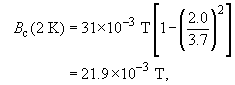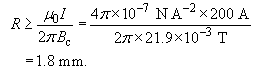Superconductivity

Start this free course now. Just create an account and sign in. Enrol and complete the course for a free statement of participation or digital badge if available.

Free course

# 2.5 Critical current

The current density for a steady current flowing along a wire in its normal state is essentially uniform over its cross-section. A consequence of this is that the magnetic field strength B within a wire of radius a, carrying current I, increases linearly with distance from the centre of the wire, and reaches a maximum value of μ﻿0I / 2﻿﻿a at the surface of the wire (see Exercise 3.6). Within a superconductor, however, the magnetic field B is zero.

## SAQ 2

What can you deduce about the current flow in a superconducting wire from the fact that B = 0 within a superconductor?

The current density within the bulk of the wire must be zero, since Ampère's law (﻿curl B = ﻿μ﻿0﻿J﻿) indicates that a non-zero current density would produce a magnetic field. The current must therefore flow in the surface of the wire.

## SAQ 3

How does the magnetic field just outside the surface of a superconducting wire, radius a, carrying current I, compare with the field just outside the surface of a normal wire with the same radius, carrying the same current?

The fields just outside the surface are identical. The currents in both wires are axially symmetric, so the integral version of Ampère's law indicates that the fields just outside the surfaces of the wires are the same.

The magnetic field strength B just outside the surface of the wire is μ﻿0﻿I / 2﻿﻿a. It follows that if the current flowing in a superconducting wire is increased, eventually the field strength at the surface of the wire will exceed B﻿c and the sample will revert to its normal state. The maximum current that a wire can carry with zero resistance is known as its critical current, and for a long straight wire the critical current I﻿c is given by I﻿c = 2﻿﻿aB﻿c / μ﻿0. A current greater than I﻿c will cause the wire to revert to its normal state. This critical current is proportional to the radius of the wire.

In the previous subsection you saw that the critical field strength is dependent on temperature, decreasing to zero as the temperature is increased to the critical temperature. This means that the superconducting current that a wire can carry will also decrease as the temperature gets closer to the critical temperature. Because of this, in real applications superconductors generally operate at temperatures less than half of the critical temperature, where the critical field strength, and therefore the critical current, is greater than 75 per cent of the maximum value.

Now, the current carried by a superconducting wire actually flows in a thin layer at the surface; it cannot be restricted to an infinitesimal layer, because that would lead to an infinite current density. As you will see in Section 3, this means that the magnetic field penetrates into this thin layer, and we derive there relationships between the field and the current density. But in the present context, the point to note is that the transition to the normal state takes place when the magnetic field strength at the surface corresponds to the critical field strength, and this occurs when the current density at the surface reaches a critical current density. This critical current density is much greater than I﻿c /﻿a﻿2 because the current flows only in a thin surface layer.

The magnetic field at the surface of a superconductor may have a contribution from an external source of magnetic field, as well as from the field produced by the current in the wire. This external field will set up screening currents in the surface layer of the material. The transition to the normal state then occurs when the vector sum of the current densities at the surface due to the current in the wire and due to the screening current exceeds the critical current density, or, equivalently, when the magnitude of the vector sum of the magnetic fields that are present at the surface of the wire exceeds the critical field strength.

## Exercise 3

Tin has T﻿c = 3.7 K and B﻿c = 31 mT at T = 0 K. What is the minimum radius required for tin wire if it is to carry a current of 200 A at T = 2.0 K﻿?

From Ampère's law, the field strength B at the surface of a wire of radius R carrying a current I is B = μ﻿0﻿I / 2﻿﻿R. From Equation 1, we also have thatso the radius R required isSMT359_1